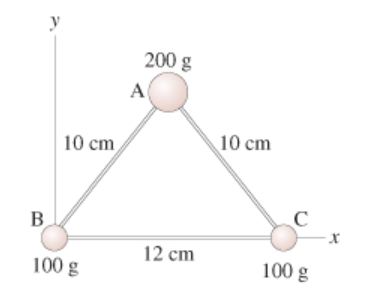# Problem: The three masses shown in the figure(Figure 1) are connected by massless, rigid rods.Part A:Find the coordinates of the center of mass. Find the x-coordinate.Part B: Find the y-coordinate.Part C:Find the moment of inertia about an axis that passes through mass A and is perpendicular to the page.Part D: Find the moment of inertia about an axis that passes through masses B and C.

###### FREE Expert Solution

x-coordinate of the center of mass;

$\overline{){{\mathbf{x}}}_{\mathbf{c}\mathbf{m}}{\mathbf{=}}\frac{{\mathbf{m}}_{\mathbf{1}}{\mathbf{x}}_{\mathbf{1}}\mathbf{+}{\mathbf{m}}_{\mathbf{2}}{\mathbf{x}}_{\mathbf{2}}\mathbf{+}\mathbf{.}\mathbf{.}\mathbf{.}\mathbf{+}{\mathbf{m}}_{\mathbf{n}}{\mathbf{x}}_{\mathbf{n}}}{{\mathbf{m}}_{\mathbf{1}}\mathbf{+}{\mathbf{m}}_{\mathbf{2}}\mathbf{+}\mathbf{.}\mathbf{.}\mathbf{.}\mathbf{+}{\mathbf{m}}_{\mathbf{n}}}}$

Similarly, y-coordinate of the center of mass:

$\overline{){{\mathbf{y}}}_{{\mathbf{cm}}}{\mathbf{=}}\frac{{\mathbf{m}}_{\mathbf{1}}{\mathbf{y}}_{\mathbf{1}}\mathbf{+}{\mathbf{m}}_{\mathbf{2}}{\mathbf{y}}_{\mathbf{2}}\mathbf{+}\mathbf{.}\mathbf{.}\mathbf{.}\mathbf{+}{\mathbf{m}}_{\mathbf{n}}{\mathbf{y}}_{\mathbf{n}}}{{\mathbf{m}}_{\mathbf{1}}\mathbf{+}{\mathbf{m}}_{\mathbf{2}}\mathbf{+}\mathbf{.}\mathbf{.}\mathbf{.}\mathbf{+}{\mathbf{m}}_{\mathbf{n}}}}$

Moment of inertia:

$\overline{){\mathbf{I}}{\mathbf{=}}{\mathbf{m}}{{\mathbf{r}}}^{{\mathbf{2}}}}$

Part A

Coordinates of the 200g mass is:

x-coordinate = 12/2 = 6 cm

y-coordinate = sqrt (102 - 62) = 8 cm.

81% (350 ratings)###### Problem Details

The three masses shown in the figure(Figure 1) are connected by massless, rigid rods.Part A:Find the coordinates of the center of mass. Find the x-coordinate.

Part B: Find the y-coordinate.

Part C:Find the moment of inertia about an axis that passes through mass A and is perpendicular to the page.

Part D: Find the moment of inertia about an axis that passes through masses B and C.